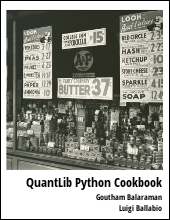This article will present an introductory example of calling lua functions from C++.

If you want to extend and customize the capabilities of a C++ application without requiring a full recompilation, then using a embedded scripting language is the way to go. Lua is one such embeddable scripting language, and is very popular among game developers. The main advantage of Lua, in my opinion, is that the core API is very minimal, has very small memory footprint. The availability of LuaJIT makes it a very performant alternative as well.

## Example Code

extern "C" {
#include "lua.h"
#include "lualib.h"
#include "lauxlib.h"
}
#include <string.h>

int main(int argc, char* argv[])
{
// initialization
lua_State * L = lua_open();
luaL_openlibs(L);

// execute script
const char lua_script[] = "function sum(a, b) return a+b; end"; // a function that returns sum of two
lua_pcall(L, 0, 0, 0);

// load the function from global
lua_getglobal(L,"sum");
if(lua_isfunction(L, -1) )
{
// push function arguments into stack
lua_pushnumber(L, 5.0);
lua_pushnumber(L, 6.0);
lua_pcall(L,2,1,0);
double sumval = 0.0;
if (!lua_isnil(L, -1))
{
sumval = lua_tonumber(L,-1);
lua_pop(L,1);
}
printf("sum=%lf\n",sumval);
}

// cleanup
lua_close(L);
return 0;
}


## Code Explained

The initial part of the code initialises the lua_State loads and executes the script with lua_pcall(L,0,0,0). Once the script is loaded, the functions are available in the global namespace. Here the script basically is a function that takes two numbers and returns the sum of the two. Using the lua_getglobal(L, "sum") call, we load the function into the stack. We can check if the function was loaded correctly using the lua_isfunction(L, -1). Then we pass the two arguments of the function by pushing them into the stack. Then the lua_pcall method executes the function and loads the result onto the stack. The successful execution of the function can be checked by checking that the stack is not nil using !lua_isnil(L,-1). The returned value can then be accessed by casting the result in the top of the stack using lua_tonumber(L,-1). We use lua_pop to clear the result from the stack.

Running this example should print:

sum=11.000000


on the screen.

## Conclusion

This article gave a very minimal example explaining how to call a lua function from C++.

lua   programming   C++

Related PostI am Goutham Balaraman, and I explore topics in quantitative finance, programming, and data science. You can follow me @gsbalaraman.

Checkout my bookUpdated posts from this blog and transcripts of Luigi's screencasts on YouTube is compiled into QuantLib Python Cookbook .